# Functions in PythonChallenge Inside! : Find out where you stand! Try quiz, solve problems & win rewards!

## Overview

A function in Python is a collection of connected statements that performs a single task. Functions help in the division of our program into smaller, modular portions. Functions help our program become more ordered and controllable as it grows in size. It also eliminates repetition and makes the code reusable.

## Scope

• Then, we will look into different types of functions.
• We will also look at the syntax and working of functions in Python.
• Then, we will understand how to call functions in Python and whether functions are passed by value or passed by reference in Python.
• We will then also understand what arguments and parameters are and categorize functions on the basis of parameters and return type.
• Then, we will learn about a few different types of functions.
• We will also learn about the scope of variables in functions in Python.
• At last, we will know the advantages of using functions in Python.

## What are Functions in Python?

Functions are blocks of code that are used to carry out various kinds of commonly done tasks. They help in ensuring the conciseness of the code by reducing data redundancy.

A function can be related to the process of making tea. If you were to make tea for the first time, your parents would have defined the steps to make it. The next time they want to drink tea, they’ll call out to you directly by saying ‘Make Tea’.

Similarly, in the case of functions, you first define the sequence of steps that you would like to carry out to achieve a certain task. Later, you can call out the Function by its name, and these steps will be performed.## Types of Functions in Python

Functions in Python can be of 2 types:

1. ### User-defined:

``````def fun():
print("Just a function having fun")
fun()
``````

Output:

``````Just a function having fun
``````
1. ### Built-in:

These are already defined within Python, and thus a user need not define them again. They can be directly used in a program or application. E.g., abs() is used to find the absolute value of a number.

``````x = abs(-7.25)
print(x)
``````

Output:

``````7.25
``````

## Rules for Naming Functions in Python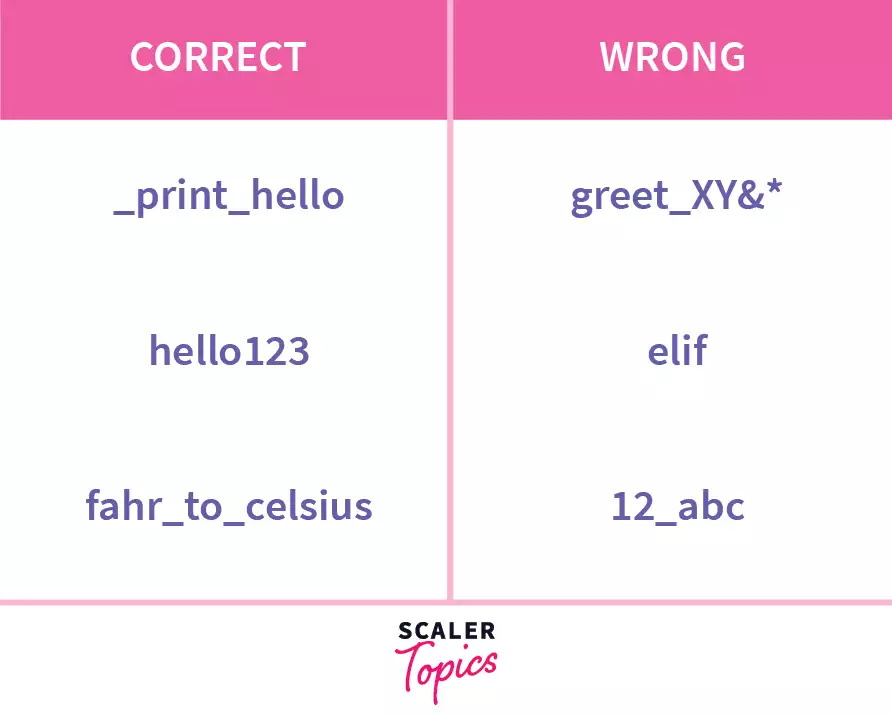If you were asked to name the process by which plants use sunlight to synthesize nutrients from CO2 and water, you wouldn’t say digestion. Similarly, a function should be named based on the task that it performs. Shorter and easy-to-understand ones are always preferred.

• They must begin with a letter or an underscore.
• They should be lowercase.
• They can be of any length.
• They shouldn’t be a keyword in python, i.e. be reserved for any purpose. To improve readability and to maintain conventions, separate words by underscores. Ensure that you give function names that define the purpose of the Function. Use camelCase only in case that is the current style of the program.

## Syntax of Python Functions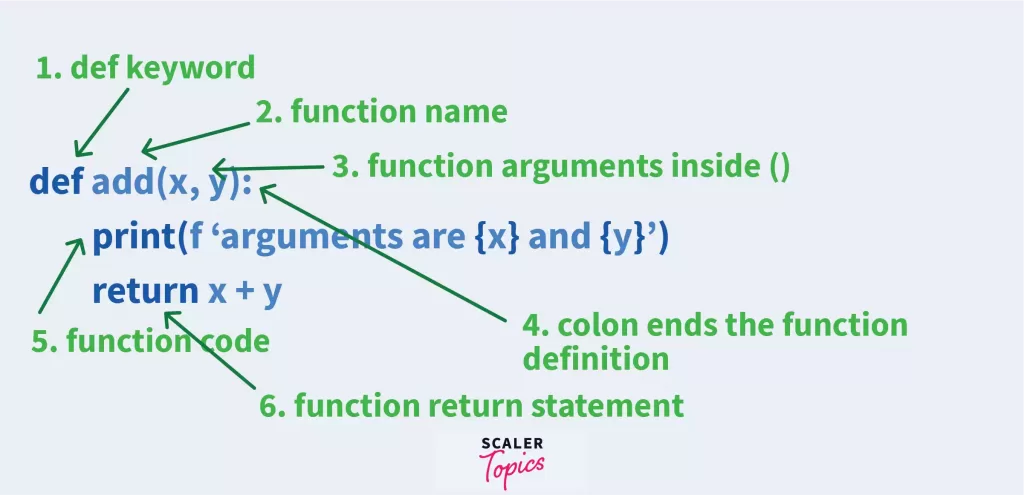``````def square(x):
"""The function returns the square of the input number"""
print("The square of "+str(x)+" is to be found.")
return x * x

print("The square is: "+str(square(10)))

``````

### Output:

``````The square of 10 is to be found.
The square is: 100
``````

The function definition consists of the name, the parameters needed, the steps that will be carried out by the Function, and returning values, if any.

Syntax:

``````def function_name (parameters):
"""docstring """
statement1
statement 2
…
…
return [expr]
``````

The components of a python function definition are:

### 1. Keyword def:

In python, the keyword def is used to define a function. It marks the beginning of the function definition.

### 2. Name of the Function:

function_name is a unique identifier that is considered the name of the Function.

### 3. Parameters:

These are the values passed to the Function. 0 or more parameters can be included in the parentheses.

### 4. Colon(:): The:

represent the start of the indented function block.

### 5. docstring:

The optional docstring or document string is used to define what the Function does.

### 6. Statements:

These include the sequence of tasks that the Function is to perform. It can also use the pass keyword in case of no statements. These statements are usually indented at the same level (usually 2 or 4 spaces).

### 7. Return Statement:

The optional return statement symbolizes the return of values from the Function to the calling code.

### Example of a function in Python

A Simple function to print Hello World on the screen.

``````def hello:
"""This function says hello to the world"""
print ("Hello World")
``````

## How Does a Python Function Work?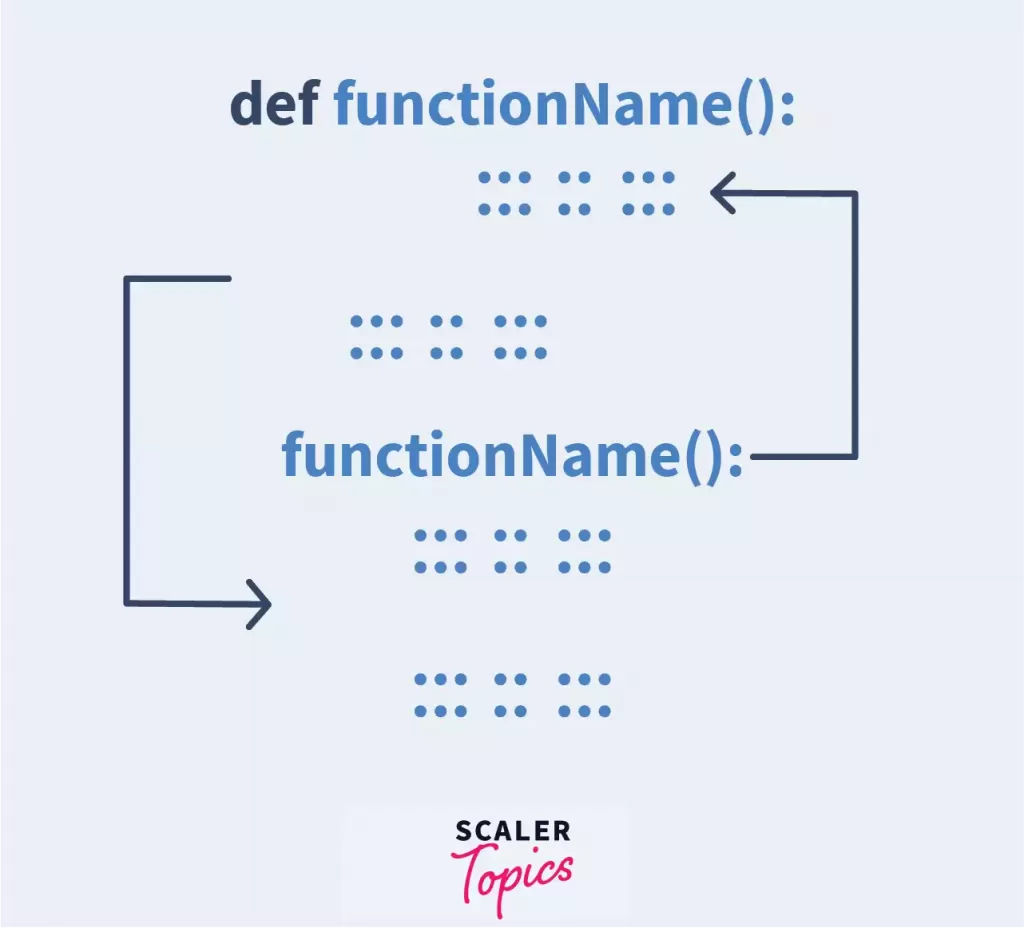A python function consists of 2 parts: Function definition and function call. To create a function in Python, you have to first define the Function, giving it a name, parameters, statements, etc., and then call it separately in the program using its names and parameters, if any.

## How to Call a Function in Python?

A function in Python is called by using its name followed by parentheses. In case parameters are present, these are included in the parentheses.

Example: To call the Function named hello, simply type the following:

``````hello()
``````

Output:

``````Hello World
``````

## Are Functions in Python Passed by Reference or Passed by Value?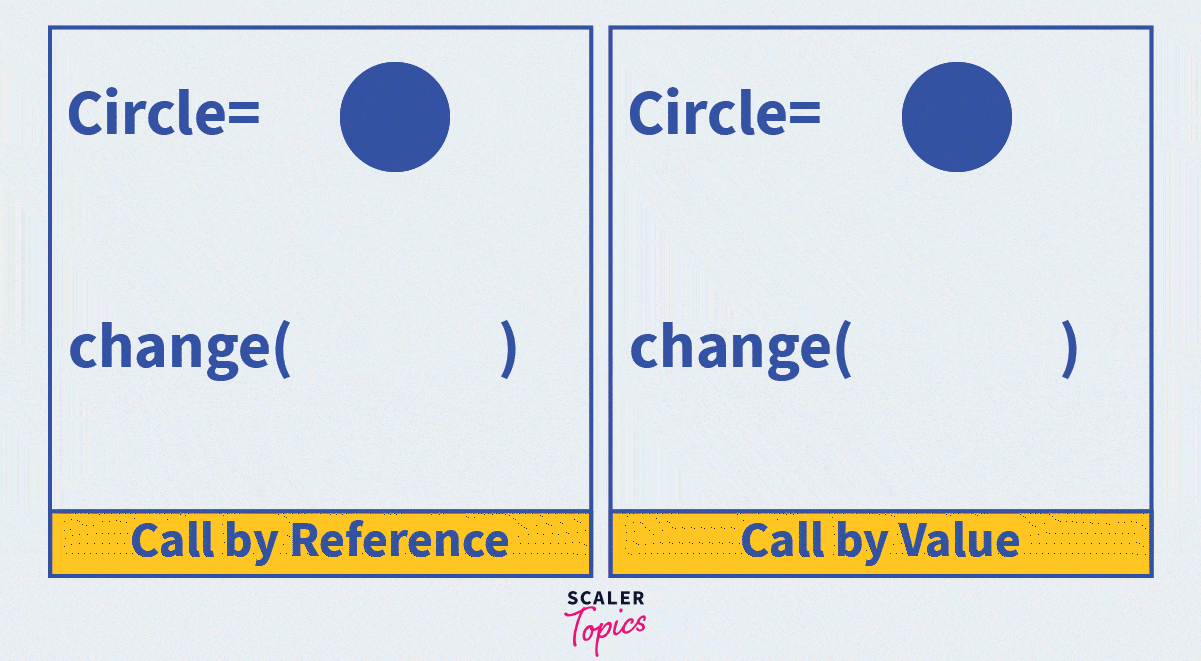Passing is the process of providing arguments (parameters) to a function. In general, parameters in functions can be passed in 2 ways: pass by reference or pass by value. In the pass-by-value, a copy of the parameter’s value is stored in the memory, and all changes are done to that. Hence, these changes aren’t reflected in the parameter in the function call. However, in pass-by reference, a copy of the address of the actual parameter is stored; thus, changes inside the Function will be reflected back in the program.

Example:

``````# Python code to demonstrate call by value
string = "Good Evening."

def greet(string):
string = "Good Morning"
print("Inside Function:", string)

greet(string)
print("Outside Function:", string)
``````

Output:

``````Inside Function: Good Morning
Outside Function: Good Evening
``````

Example:

``````# Python code to demonstrate call by reference

def append_to_list(list1):
list1.append(35)
print("Inside Function: ", list1)

multiples_of_5 = [5,10,15,20,25,30]
append_to_list(multiples_of_5)
print("Outside Function: ", multiples_of_5)
``````

Output:

``````Inside Function: [5, 10, 15, 20, 25, 30]
Outside Function: [5, 10, 15, 20, 25, 30]
``````

Consider the following example where i is passed from main() to inc(). The inc() function serves the purpose of incrementing the value being sent from main by 1. id() is a built-in function in Python that gives memory addresses of objects being passed.

``````def main():
i = 5
print(f"Initial value of i in main: {i}")
print(f"Initial address of i in main: {id(i)}")
inc(i)
print(f"Final value of i in main:{i}")
print(f"Final address of i in main: {id(i)}")

def inc(n):
print(f"Initial value of n in inc: {n}")
print(f"Initial address of n in inc: {id(n)}")
n = n+1
print(f"Final value of n in inc: {n}")
print(f"Final address of n in inc: {id(n)}")

main()
``````

Output:

``````Initial value of i in main: 5
Initial address of i in main: 140705849923360
Initial value of n in inc: 5
Initial address of n in inc: 140705849923360
Final value of n in inc:6
Final address of n in inc: 140705849923392
Final value of i in main:5
Final address of i in main: 140705849923360
``````

From the above example, we can draw the following conclusion:

• The address of i remains constant.
• The initial address of n is equal to that of i.
• The address of n changes on reassignment of value

Although the fact that the incrementing doesn’t reflect in the main() suggests that the Python code uses a pass-by-value mechanism, the 2nd conclusion clearly proves that this isn’t a case of pass-by-value. Thus, we can say that Python uses a mechanism that is different from a pass-by-value or pass-by-reference.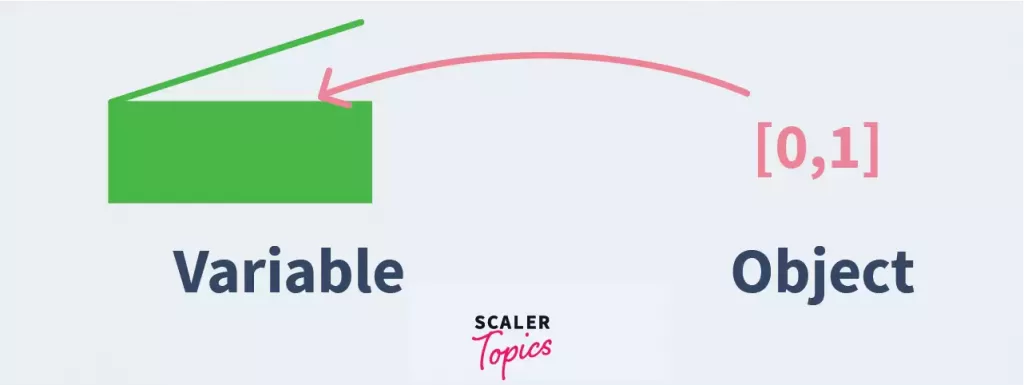Unlike languages that use pass by value or pass by reference, python uses a mechanism known as “Pass by Object Reference”. Here, the name (aka object reference) is passed. A variable is assumed to consist of an object. Objects in python are of 2 types: Mutable and Immutable. An immutable object is one whose values cannot be changed once created. E.g., Strings, tuple. A Mutable object is one whose values can be changed. E.g., Sets, List. If the object under consideration is mutable, the object modifications will persist and will not in the case of immutable objects.

Example: Function that helps to implement a change in value using functions.

``````def inc(n): # Accept an argument, return a value.
return n+1
x = 4
x = inc(x) #reassign the value
print(x)
``````

Output:

``````5
``````

## Types of Arguments in Python

Functions with arguments/parameters are called parameterized functions. To call these functions, the number and order of arguments should be as given in the function definition.

A function can have 1 or more parameters. A function in python can accept up to 255 parameters.

The arguments/parameters used in the python function definition are called formal arguments or formal parameters while the ones in the function call are called local arguments or local parameters. The name is based on their location in the program.

Function parameters can have their type defined along with them, as given in the example:

``````def multiply(a:int, b:int):
print (a*b)
multiply(5,10)
``````

Output:

``````50
``````

Example: Function with Single Parameter

``````def hello(name):
print ("Hello", name)
hello("Anna")
``````

Output:

``````Hello Anna
``````

Example: Function with Multiple Parameters

``````def hello(name1, name2, name3):
print("Hello", name1, "\nHi", name2, "\nHola", name3)
``````

Output:

``````Hello Sailee
Hi Kanchan
``````

There are four major types of arguments:

• Required arguments
• Keyword arguments
• Default arguments
• Variable-length arguments

### Required Arguments

In such functions, the exact number of arguments are required to be passed in the order in which it is defined in the Function.

Example:

``````def hello(name):
print ("Hello", name)
hello("Anna")
``````

Output:

Hello Anna

``````hello()
``````

Output:

``````Traceback (most recent call last):
File "C:\Users\user\Desktop\test.py", line 3, in <module>
hello()
TypeError: hello() missing 1 required positional argument: 'name'
``````

In the above example, the hello() function requires exactly one argument (name) to be passed in the function call, without which it will give an error.

### Keyword Arguments(**kwargs)

Usually, when you call a function using arguments, these are passed to the function definition in the order in which they are passed in. For example:

``````def hello(fname, lname):
print('Hello', fname, lname)
hello('Murthy','Sunny')
``````

In the example above, fname takes the value Murthy and lname takes the value Sunny. There might be times when you don’t know the order in which the arguments are present in the Function and thus want to use them in the function call in a different order. This is exactly where Keyword Arguments come in. Using keyword arguments, a function can be called by providing parameter values in any order, as long as the parameter names are mentioned along with them.

Example:

``````def hello(fname, lname):
print('Hello', fname, lname)

hello(lname='Murthy', fname='Sunny')
``````

Output:

``````Hello Sunny Murthy
``````

What if you don’t know the number of arguments being passed? Functions can have single parameters prefixed with ** to map a variable number of keyword arguments in different order.

``````def hello(**name):
print("Hello", name['fname'], name['lname'])

hello(fname="Anne", lname="Sullivan")
hello(lname="Pichai", fname="Sundar")
hello(fname="Narendra", mname="Damodar", lname="Modi")
hello(fname="Bill") #gives error
``````

Output:

``````Hello Anne Sullivan
Hello Sundar Pichai
Hello Narendra Modi
``````

### Default Arguments

The formal parameters can be assigned default values as a failsafe, in case a local value isn’t passed. In such cases, the default value will be used inside the Function.

Example:

``````def hello (name="Anna"):
print("Hello", name)
hello()
hello("Rohan")
``````

Output:

``````Hello Anna
Hello Rohan
``````

### Variable-Length Arguments

Not always will you know the number of arguments that can be passed to the Function. If the number of arguments passed is unknown, you can add an asterisk (*) before the parameter in the function definition.

Example:

``````def hello(*names):
i=0
print("Hello")
for name in names:
print(name)
hello("Anna", "Ema", "Sarah")
``````

Output:

``````Hello
Anna
Emma
Sarah
``````

## Categorizing Functions in Python based on Parameters and Return Value

Based on Function Definition, a function can be categorized into 4 types:

### a) Without parameters, without return statement

Syntax:

``````def function_name():
statements
``````

Example:

``````def hello():
print("Hello World!")
hello()
``````

Output:

``````Hello World
``````

### b) With Parameters, without return statement

Syntax:

``````def function_name(argument):
statements
``````

Example:

``````def hello(fname):
print("Hello " + fname)
hello("Tina")
``````

Output:

``````Hello Tina
``````

### c) Without parameters, with the return statement

Syntax:

``````def function_name():
statements
return [arg]
``````

Example:

``````def isEven(x):
if (x % 2 == 0):
return "Even"
else:
return "Not Even"

even10 = isEven(10)
print(even10)
``````

Output:

``````Even
``````

### d) With parameters, with return statement

Syntax:

``````def function_name(argument):
statements
return [arg]
``````

Example:

``````def sum(a, b):
return a + b
total=sum(10,20)
print(total)
``````

Output:

``````30
``````

## Recursive Functions in Python

Recursion is the process in which a function calls itself. Recursive functions are used mostly for sequence generation and are used to make the code look clean and elegant.

Example of a function to calculate the sum of n consecutive natural numbers recursively:

``````def rec_sum(n):
if n<=1:
return n
else:
return n + rec_sum(n-1)
y = rec_sum(5)
print(y)
``````

Output:

``````15
``````

## Anonymous Functions in Python

An anonymous in python is a function that is defined without a name. Unlike functions defined using the def keyword, these are defined using the lambda keyword and are hence called lambda functions. They can have 0 or more arguments but only one return value.

Syntax:

``````lambda arguments: expression
``````

Example:

``````square = lambda x: x * x
print(square(2))
``````

The above example as a normally defined function could be written as:

``````def square(x):
return x * 2
print(square(2))
``````

Output:

``````4
``````

## Scope of Variables in Python

### Global Variables

Any variable declared outside the Function is called a global variable. Such a variable has global scope, i.e., it is accessible everywhere, whether inside or outside of the Function.

Example:

``````x = 5

def f1():
print("x in =", x)

f1()
print("x out=", x)
``````

Output:

``````x in =5
x out=5
``````

### Local Variables

A variable declared inside the Function’s body is called a local variable. Such a variable is said to have a local scope, i.e., it is only accessible within the Function and not outside.

Example:

``````def f2():
y = 3

f2()
print(y)
``````

Output:

``````NameError: name 'y' is not defined
``````

Example: Global and Local Variables with the Same Name

``````x = 15

def f4():
x = 200
print("Local x:", x)

f4()
print("Global x:", x)
``````

Output:

``````Local x: 200
Global x: 15
``````

## Pass Statement

Empty functions are used as a means of time delay. Since functions cannot be empty, the pass statement can be used to avoid errors caused by a lack of statements.

Example:

``````def function1():
pass
``````

## Advantages of Functions in Python

Helps in increasing modularity of code – Python functions help divide the code into smaller problems and solve them individually, thus making it easier to code.

Minimizes Redundancy – Python functions help you save the effort of rewriting the whole code. All you got to do is call the Function once it is defined.

Maximes Code Reusability – Once defined, the python Function can be called as many times as needed, thus enhancing code reusability.

Improves Clarity of Code – As the large program is divided into sections with the help of functions, it helps in increasing the readability of code, at the same time ensuring easy debugging.

## Conclusion

Let's recall functions in Python in a nutshell:

• Functions are used to reduce repetition and make the code more modular.
• Python neither uses pass by value nor pass by reference mechanism, but it uses pass by object reference sort of mechanism.
• We can pass up to 255 parameters in a function in Python.
• Scope of a variable in a function can be changed from local to global by using the global keyword.
• Pass statement can be used to write an empty function.
Challenge Time!Time to test your skills and win rewards! Note: Rewards will be credited after the next product update.Certificates
Python TutorialCriteria
You’ll be able to claim a certificate for any course you have access to only after you’ve spent enough time learning. The time required is determined by the length of the course.
Certificates are a fantastic way to showcase your hard-earned skills to employers, universities, and anyone else that may be interested. They provide tangible proof that you’ve completed a course on Scaler Topics. In many cases they can help you claim a training reimbursement or get university credit for a course.